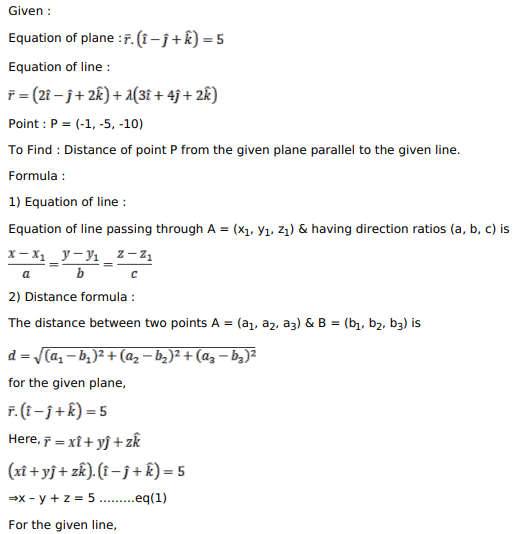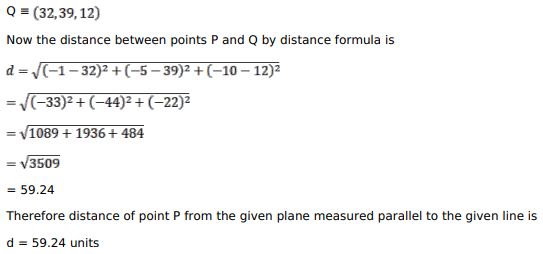# Solve this followingQuestion:

Find the distance of the point $\mathrm{A}(-1,-5,-10)$ from the point of intersection of the line $\vec{r}=(2 \hat{i}-\hat{j}+2 \hat{k})+\lambda(3 \hat{i}+4 \hat{j}+2 \hat{k})$ and the plane $\vec{r} \cdot(\hat{i}-\hat{j}+\hat{k})=5$.

HINT: Convert the equations of the line and the plane to Cartesian form.

Solution: